Video Tutorial Bond Energies and Enthalpies

Quick Notes Bond Enthalpies

• A bond enthalpy refers to the enthalpy change (ΔH) that occurs when one moles worth of a particular bond type is broken (in gaseous phase).
• Energy is released by two atoms when a bond is formed between them. Bond making is exothermic (-ΔH).
• That same amount of energy is absorbed by the two atoms when the bond is broken. Bond breaking is endothermic (+ΔH).
• The overall enthalpy change that occurs in a reaction can be found by subtracting the sum of all the bond enthalpies of bonds formed (in products) from the sum of all bond enthalpies of bonds broken (in reactants).
•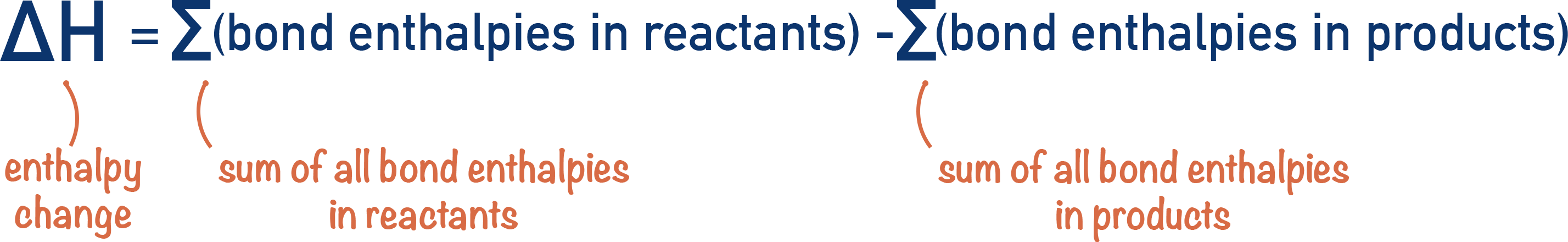• Mean bond enthalpies are used to account for the different environments a bond might be in.

Full Notes Bond Enthalpies

When a bond is made between two atoms (atomic bond), the overall energy of the two atoms lowers – they are more stable when bonded. This means energy is released by two atoms as they form a bond - bond forming is an exothermic process.To break an atomic bond, we have to give the bonded atoms energy so they can ‘overcome’ the forces of attraction in the bond and break it. This means atoms gain energy as a bond breaks and they become less stable - bond breaking is an endothermic process.Energy is required to break a bond; energy is released when a bond is formed.

Every bond requires different amounts of energy to break, so it releases different amounts of energy when it’s formed.

A bond enthalpy refers to the enthalpy change (ΔH) that occurs when one moles worth of a particular bond type is broken (in gaseous phase).

## Mean Bond Enthalpies

The exact environment of a bond has an influence on its bond enthalpy. For example, a carbon-hydrogen bond in methane will have a slightly different bond enthalpy compared to a carbon-hydrogen bond in methanoic acid.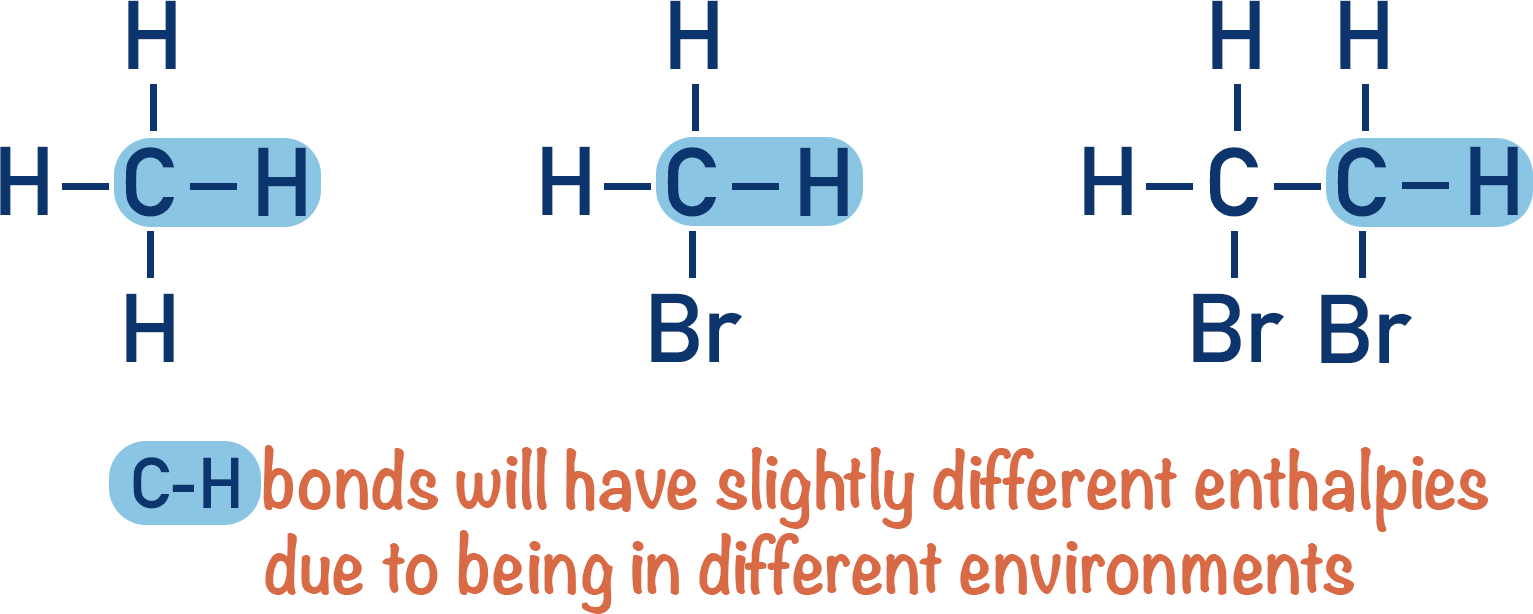It’s not realistic to use specific bond enthalpies for specific molecules, instead we use mean bond enthalpies. A mean bond enthalpy is just an average value for a bond enthalpy based on data from a range of different compounds containing that bond type.

The use of mean bond enthalpies to find enthalpy changes for a reaction (see below) gives values that may differ from experimental results.

## Using Bond Enthalpies (to find enthalpy change, ΔH)

The enthalpy change (ΔH) that occurs for a reaction is based on the energy needed to break bonds in the reactants and the energy released when bonds are formed to make the products.

We can use the sum of bond enthalpies in reactants and products to find the enthalpy change for a reaction. The enthalpy change is essentially the difference between the two.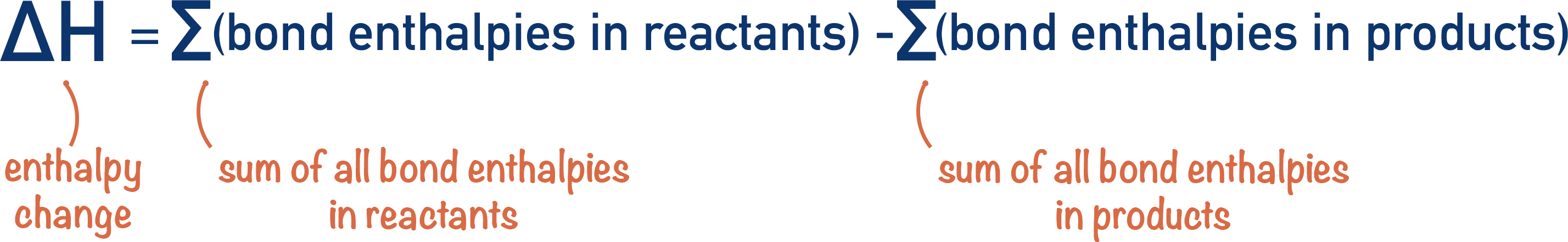For Example...

In the combustion of propane, C3H8, all carbon-carbon and carbon-hydrogen bonds in propane must be broken before the products (carbon dioxide and water) can be formed. Bonds between oxygen atoms in the 5 O2 molecules must also be broken.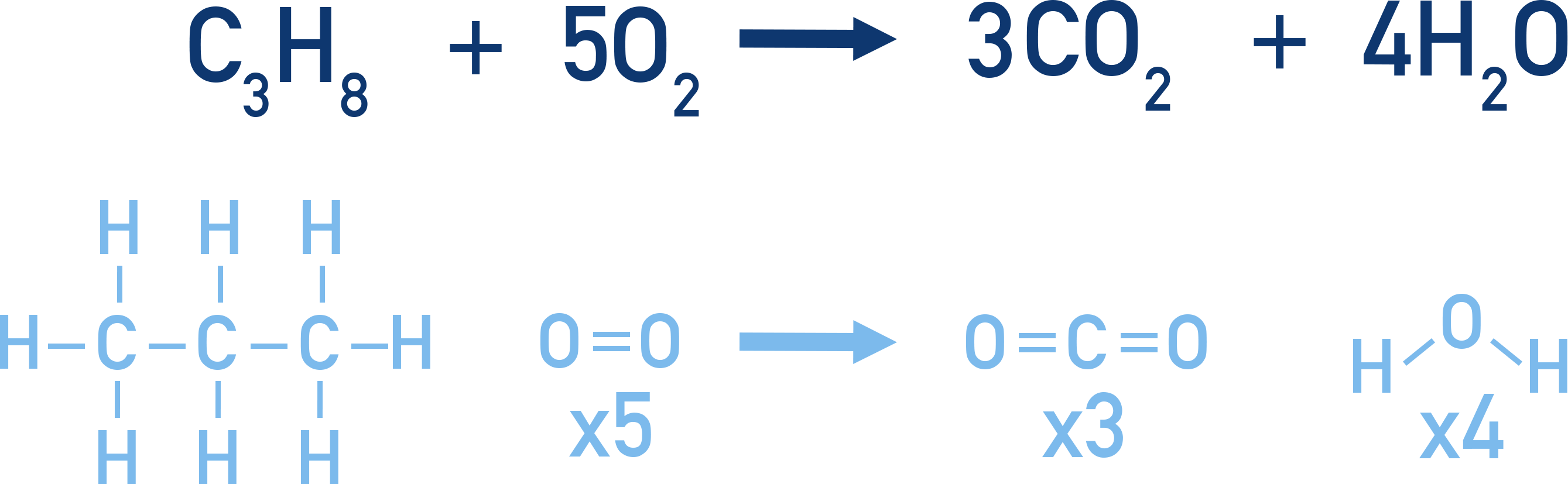By using bond enthalpy data, we can see how much energy is needed to break all these bonds (per mol of propane).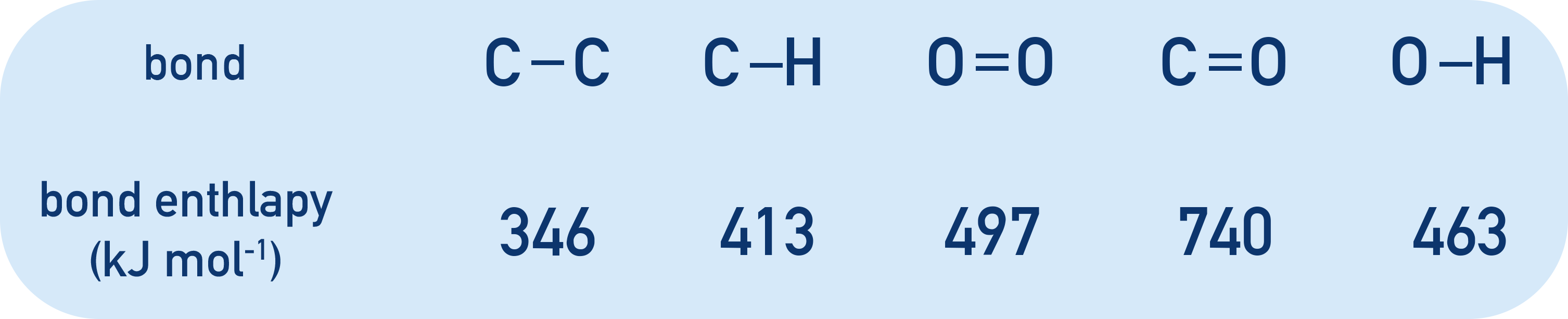In 1 mole of C3H8, there are 2 C-C bonds and 8 C-H bonds. For 5 moles of O2, there are 5 moles of O=O bonds.

C-C bond enthalpy = 346 kJmol-1. 2 x 346 = 692 kJmol-1.

C-H bond enthalpy = 413 kJmol-1. 8 x 413 = 3304 kJmol-1.

O=O bond enthalpy = 497 kJmol-1. 5 x 497 = 2485 kJmol-1.

Sum of all bond enthalpies in reactants = 6481 kJmol-1.

1 mole of C3H3 forms 3 moles of CO2 and 4 moles of H2O.

3 moles of CO2 contains 6 C=O bonds and 4 moles of H2O contains 8 O-H bonds.

C=O bond enthalpy = 740 kJmol-1. 6 x 740 = 4440 kJmol-1.

O-H bond enthalpy = 463 kJmol-1. 8 x 463 = 3704 kJmol-1.

Sum of all bond enthalpies in products = 8144 kJmol-1.

This means 6481 kJ of energy is needed to break all bonds and then 8144 kJ of energy is released when new bonds are formed (per mol of C3H8). The overal enthalpy change, ΔH, will be the difference between the two.ΔH = (6481) - (8144) = -1663 kJmol-1

Note that is is a negative value for ΔH as energy is released overall for the reaction, an exothermic reaction. More energy must be giving out during bond formation than is required to break bonds at the start.